Study Guide

# Rational Functions - Adding and Subtracting Rational Expressions

## Adding and Subtracting Rational Expressions

Remember fractions? They're about to rear their ugly heads again in the world of rational expressions. Rational expressions behave just like fractions, except they're more ornery. (It's those extra elements like variables ad nauseam that complicate things.)

To add or subtract a couple of rational expressions, we haul everything into the numerator and find one common denominator. The steps are painless, usually. If necessary, we can factor each denominator and find the LCM (Least Common Multiple).

Then, the LCM stands in for our common denominator. Next, we add up the numerators and put that result on top of the common denominator.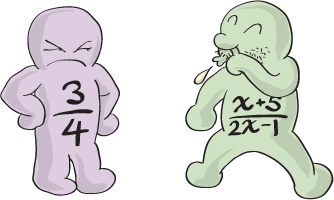### Sample Problem

Add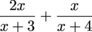.

First, we need a common denominator.

The LCM of (x + 3) and (x + 4) is (x + 3)(x + 4). In other words, (x + 3)(x + 4) is the "lowest" expression that's divisible by both (x + 3) and (x + 4).

Next, we need to multiply each term by a ratio of 1 that will put it over our LCM.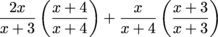Simplify the numerator and put the whole thing over the common denominator.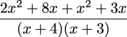Collect like terms in the numerator.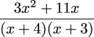Factor the x out of the numerator.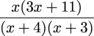There are no common factors between the numerator and the denominator, so the answer is: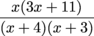### Sample Problem

Subtract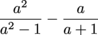.

Let's factor that first denominator.

a2 – 1 = (a + 1)(a – 1)

We want the LCM of (a + 1)(a – 1) and (a + 1). What's the lowest expression that's evenly divisible by both denominators?

When there are common factors in our denominators (like (a + 1) this time around), one sweet trick to finding the LCM is to take the highest power of each factor and multiply 'em together. So, since we've got that (a + 1) in both denominators, we'll take (a + 1) to the first power, or just (a + 1). We don't need two copies of (a + 1), since it shows up in each fraction once. We'll also take (a – 1) to the first power.

Boom: the LCM is (a + 1)(a – 1).

Next, we multiply the second term by a ratio of 1 to get it over our LCM. (The first fraction already has the LCM as its denominator, so it gets to stay just like it is.)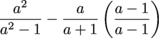Simplify the numerator and put it all over the LCM.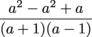Finish up by collecting like terms.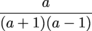### Sample Problem

Add.

First we factor the denominators.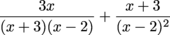Now take the highest power of each factor to get the LCM. There's only one (x + 3), so take that to the first power. There's a (x – 2) in the first fraction and a (x – 2)2 in the second fraction, so take that factor to the second power. Our LCM is (x + 3)(x – 2)2.

Now we multiply by the correct ratios of 1.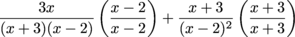Simplify and throw it all over the LCM.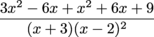Collect like terms in the numerator.There you have it: one piping-hot sum.